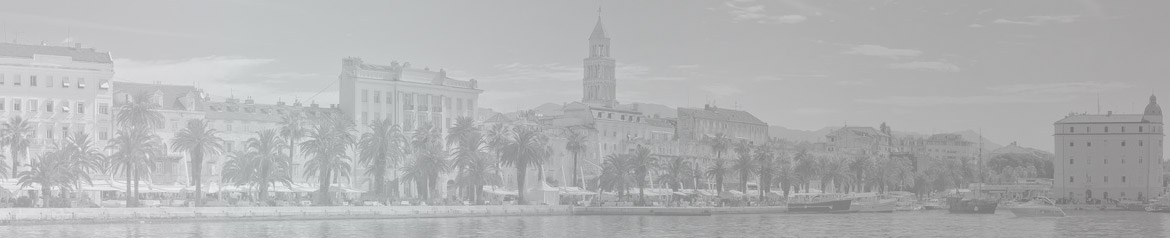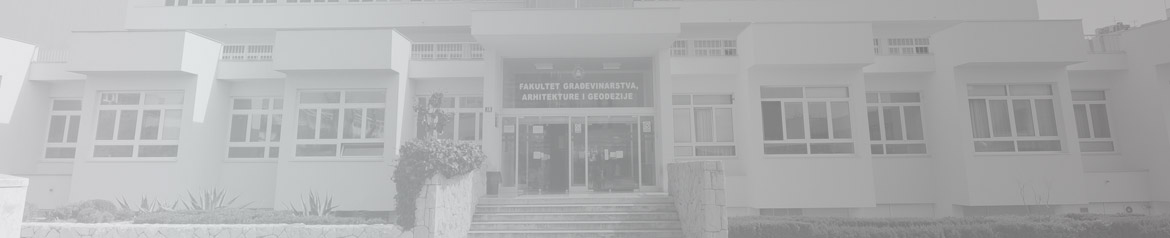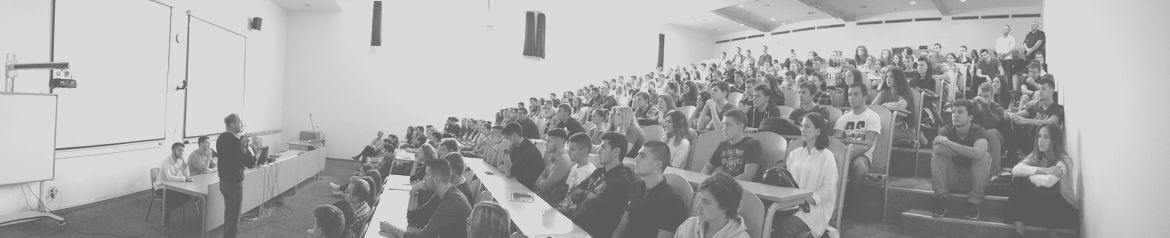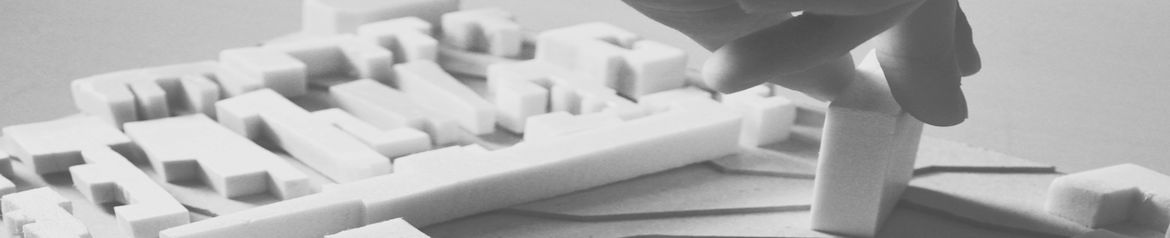•••••# Sveučilište u Splitu - Fakultet građevinarstva, arhitekture i geodezijeUniversity of Split - Faculty of Civil Engineering, Architecture and Geodesy

## Department of Theory of Structures

 Study Course Semester Hours ECTS Undergraduate University Study of Civil Engineering Mechanics I Building Statics I Building Statics II III. II. IV. 30+45 30+30 45+30 6,0 5,0 6,0 Graduate University Study of Civil Engineering Dynamics of structures and earthquake engineering Stability of structures Masonry structures Nonlinear building statics Dynamic models of earthquake engineering Building physics Design of structures by computer I. I. III. III. II. II. I. 30+15 30+15 30+30 30+30 30+30 30+30 30+30 4,0 5,0 5,0 5,0 5,0 5,0 5,0 Postgraduate Doctoral Study of Civil Engineering Selected chapters of dynamics of structures and earthquake engineering Selected chapters of stability of structures Finite element method Information engineering Engineering simulation techniques 6,0 6,0 6,0 6,0 6,0 Undergraduate University Study of Architecture and Urban Planning Basis of structures I Basis of structures II I. II. 30+30 30+30 6,0 6,0 Undergraduate Professional Study of Civil Engineering Designing and analysing structures by computer Building physics Masonry structures Building regulations Execution of building works III. V. V. II. IV. 30+30 15+15 30+30 30+00 50+10 5,0 2,0 5,0 3,0 5,0

Learning Outcomes:

Undergraduate University Study of Civil Engineering
Mechanics I
- a student will be able to:
- analyze statical determinacy of the plane and spacial structural systems;
- analyze and calculate support and internal reactions of the plane and spacial structural systems;
- analyze and solve dry friction problems;
- calculate internal forces in the members of statically determinante plane and spatial trusses;
- calculate internal cross-section forces and draw internal forces diagrams of statically determinate plane and spacial beams;
- analyse equilibrium of cables under distributed and concentraced loads and calculate their internal forces;
- apply the method of virtual work and potential energy in analysis of simple line structures.

Building Statics I - a student will be able to:
- determine the kinematic and static stability of plane and spatial structures;
- recalculate internal forces in statically determinate and indeterminate plane and spatial trusses;
- recalculate internal forces in statically determinate plane and spatial beam structures;
- calculate the displacements of nodes in beam and truss structures;
- construe influential lines of statically determinate plane beams;
- construe influential lines of statically determinate plane truss structures;
- construe an envelope to the effect of the multiple loads on the beams.

Building Statics II - a student will be able to:
- distinguish the basic types of deformation of the beam carrier in the space;
- recalculate the internal forces in statically in determinate line element by finite element method;
- recalculate the internal forces in statically in determinate line element by force method;
- formulate the procedure for analysis of beam structures by finite element method;
- interpret the responses plates, plates on elastic fundations, panels and walls with openings;
- model and interpret responses from columns, panels and supporting walls;
- comment on static modeling and computer error.

Graduate University Study of Civil Engineering
Dynamics of Structures and Earthquake Engineering
- a student will be able to:
- solve response of single-degree-of-freedom systems in the time and frequency domain;
- solve response of multi-degree-of-freedom systems by modal analysis;
- calculate response of civil engineering structures under earthquakes by lateral force method and modal response spectrum analysis;
- recalculate the dimension of simple civil engineering structures according to capacity design and structural regulations (Eurocode 8) for design of earthquake resistant structures;
- design of earthquake resistant structures.

Stability of Structures - a student will be able to:
- determine problems of stability of building structures;
- analyse and solve stability problems of linear structures;
- analyse and solve stability problems of surface structures;
- analyse non-linear material and non-linear geometrical linear structures.

Masonry Structures - a student will be able to:
- recalculate the structure of a masonry building;
- to conceive the construction concept of the earthquake-resistant structure;
- construct details of masonry structures;
- design the interlayer masonry structures;
- use regulations and standards for the calculation of masonry structures.

Nonlinear Building Statics - a student will be able to:
- carry out the material and geometric nonlinear calculation of reinforced-concrete, metal and wooden frame structures and evaluate their behaviour;
- recalculate the mechanical resistance and deformability of reinforced-concrete, metal and wooden structures based on the push-over analysis;
- recalculate the behaviour of structures on the basis of non-linear bearing support and base substrate;
- create and evaluate, based on geometric nonlinear calculation, the behaviour of deformable structures from ropes and platens;
- create and evaluate, based on the nonlinear calculation, the behaviour of r-c plates and shells.

Dynamic Models of Earthquake Engineering - a student will be able to:
- calculate linear response of civil engineering structures under earthquakes by modal response spectrum analysis;
- calculate non-linear response of civil engineering structures under earthquakes by non-linear static (pushover) analysis and non-linear dynamic (time-history) analysis;
- recalculate the dimension of reinforced-concrete structures according to capacity design and detailing rules defined by structural regulations (Eurocode 8);
- estimate the influence of seismic isolation to the behaviour of the structures under the earthquake;
- design of earthquake resistant structures;
- validate the behaviour of civil engineering structures under the earthquake.

Building Physics- a student will be able to:
- design a concept of thermal protection and noise protection in buildings;
- design layers of structures from the point of view of thermal protection and noise protection;
- calculate thermal losses through building structure;
- calculate sound insulation of the barrier from the air noise and the sound level value of impact;
- predict noise protection measures.

Design of Structures by Computer - a student will be able to:
- create spatial computer geometric models;
- create computer models of line structures and evaluate their responses;
- create computer models of surface structures and evaluate their responses;
- calculate complex structures under earthquake excitation;
- write a program in FORTRAN.

Postgraduate Doctoral Study of Civil Engineering
Selected Chapters of Dynamics of Structures and Earthquake Engineering - a student will be able to:
- create non-linear deterministic model of the dynamics of structures;
- analyse an earthquake resistance of the structures by a non-linear static (pushover) analysis;
- formulate the models of direct response of the structures subjected to earthquake exitation;
- formulate stohastic models of the dynamics of structures;
- model soil-structure interaction in dynamic problems.

Selected Chapters of Stability of Structures - a student will be able to:
- create numerical models of material and geometric nonlinear bearing and stability of spatial line structures;
- model the problems of bending, lateral and torsional stability of the spatial frame structures;
- create numerical models of bearing capacity and stability of slabs and shells according to the theory of small and large displacements;
- analyze the bearing capacity spectrum of the pressure flexure elements and apply quasi-nonlinear methods.

Finite Element Method - a student will be able to:
- develop mathematical and numerical formulation for finite element analysis of solids and structures;
- produce computational programme based on finite element method;
- estimate solution accuracy of numerical models;
- make judgement on aplicabillity of used numerical procedure in analysis of the problem;
- choose and recommend a suitable numerical formulation and model for solving the problem between multiple variants and to defend attitude.

Information Engineering - a student will be able to:
- distinguish programming languages;
- develop an engineering application for an engineering process;
- assess advantages of structured and object-oriented programming approach;
- develop graphic interface for application;
- develop teamwork skills, develop skills for space distributed development, parallel and distributed computing and intelligent engineering.

Engineering Simulation Techniques - a student will be able to:
- integrate formulation of large displacements and rotations into finite element method;
- formulate modern methods of engineering simulations;
- present scientific publications with usage of modern engineering notation;
- formulate processes of contact interaction and fragmentation in discrete systems.

Undergraduate University Study of Architecture and Urban Planning
Basis of Structures I
- a student will be able to:
- distinguish types of structures;
- analyze statical determinacy of the structural systems;
- analyze and calculate support and internal reactions of the plane structural systems;
- calculate internal forces in the members of statically determinante plane trusses;
- calculate internal cross-section forces and draw internal forces diagrams of statically determinate simple and composite plane beams.

Basis of Structures II - a student will be able to:
- calculate stresses and deformations in simple structures exposed to longitudinal and lateral deformation, bending and torsion;
- dimension simple line structures;
- calculate the deformation line of the girder;
- calculate simple statically indeterminate line structures using the force method and displacement method.

Undergraduate Professional Study of Civil Engineering
Designing and Analysing Structures by Computer
- a student will be able to:
- make construction drawings using computer programs;
- apply procedures for automated drawing and design of computer designs;
- create computer models and interpret the results on plane truss structures;
- create computer models and interpret the results on plane beam structures.

Building Physics - a student will be able to:
- analyze and recalculate the composition of constructive building elements, taking into account thermal protection, protection against water vapor diffusion, thermal stability and noise protection;
- distinguish thermal insulating materials considering their thermal characteristics and place of instalation;
- identify causes and the consequences of thermal bridges in the building;
- use thermal protection and noise protection studies in the design and construction phase.

Masonry Structures - a student will be able to:
- construct the appropriate constructive system of the masonry structure depending on the type of building;
- conceive a calculation model of a masonry structure;
- verify the bearing capacity of the masonry structures according to the method of the boundary states;
- shape the details of the elements of the masonry structure.

Building Regulations - a student will be able to:
- apply applicable regulations in the area of construction and regulations in the process of adoption;
- carry out the process of obtaining and using documents in the process of construction and spatial planning.

Execution of Building Works - a student will be able to:
- cooperate in the management and organization of construction sites;
- elaborate building technologies;
- perform works and buildings of less complexity.